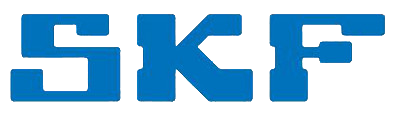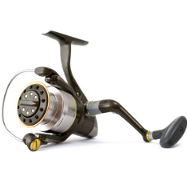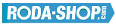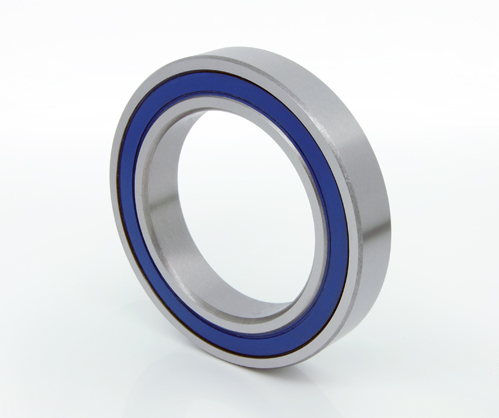# Fishing Reel BearingsManufacturer Product Image Item Name Price### 606-2RS

Miniature Metric Series Chrome Steel Rubber Seals on Both sides Dimension d=6.00 Dimension D=17.00 Dimension B=6.00 Dinamic Load Rating=2263 Static Load Rating=846 Weight=0.007gr. Speed limit Grease=38.0 Speed limit Oil=45.0
inside diameter = 6.00
outside diameter = 17.00
width = 6.00
€2.24### 608-2RS

Miniature Metric Series Chrome Steel Rubber Seals on Both sides Dimension d=8.00 Dimension D=22.00 Dimension B=7.00 Dinamic Load Rating=3293 Static Load Rating=1379 Weight=0.014gr. Speed limit Grease=34.0 Speed limit Oil=40.0
inside diameter = 8.00
outside diameter = 22.00
width = 7.00
€2.24### 608-2Z

Miniature Metric Series Chrome Steel Metallic Shields on both sides Dimension d=8.00 Dimension D=22.00 Dimension B=7.00 Dinamic Load Rating=3293 Static Load Rating=1379 Weight=0.014gr. Speed limit Grease=34.0 Speed limit Oil=40.0
inside diameter = 8.00
outside diameter = 22.00
width = 7.00
€2.24### 608-2ZW6

Miniature Metric Series Chrome Steel Metallic Shields on both sides Dimension d=8.00 Dimension D=22.00 Dimension B=6.00 Dinamic Load Rating=3293 Static Load Rating=1379 Weight=0.013gr. Speed limit Grease=34.0 Speed limit Oil=40.0
inside diameter = 8.00
outside diameter = 22.00
width = 6.00
€2.24### MR106-2RS

Miniature Metric Series Chrome Steel Rubber Seals on Both sides Dimension d=6.00 Dimension D=10.00 Dimension B=3.00 Dinamic Load Rating=496 Static Load Rating=218 Weight=0.001gr. Speed limit Grease=45.0 Speed limit Oil=53.0
inside diameter = 6.00
outside diameter = 10.00
width = 3.00
€2.24### MR106-2Z

Miniature Metric Series Chrome Steel Metallic Shields on both sides Dimension d=6.00 Dimension D=10.00 Dimension B=3.00 Dinamic Load Rating=496 Static Load Rating=218 Weight=0.001gr. Speed limit Grease=45.0 Speed limit Oil=53.0
inside diameter = 6.00
outside diameter = 10.00
width = 3.00
€2.24### MR126-2RS

Miniature Metric Series Chrome Steel Rubber Seals on Both sides Dimension d=6.00 Dimension D=12.00 Dimension B=4.00 Dinamic Load Rating=716 Static Load Rating=295 Weight=0.002gr. Speed limit Grease=43.0 Speed limit Oil=50.0
inside diameter = 6.00
outside diameter = 12.00
width = 4.00
€2.24### MR126-2Z

Miniature Metric Series Chrome Steel Metallic Shields on both sides Dimension d=6.00 Dimension D=12.00 Dimension B=4.00 Dinamic Load Rating=716 Static Load Rating=295 Weight=0.002gr. Speed limit Grease=43.0 Speed limit Oil=50.0
inside diameter = 6.00
outside diameter = 12.00
width = 4.00
€2.24### S604-2RS

Miniature Metric Series Stainless Steel Rubber Seals on Both sides Dimension d=4.00 Dimension D=12.00 Dimension B=4.00 Dinamic Load Rating=957 Static Load Rating=350 Weight=0.003gr. Speed limit Grease=48.0 Speed limit Oil=56.0
inside diameter = 4.00
outside diameter = 12.00
width = 4.00
€3.93### S604-2Z

Miniature Metric Series Stainless Steel Metallic Shields on both sides Dimension d=4.00 Dimension D=12.00 Dimension B=4.00 Dinamic Load Rating=957 Static Load Rating=350 Weight=0.003gr. Speed limit Grease=48.0 Speed limit Oil=56.0
inside diameter = 4.00
outside diameter = 12.00
width = 4.00
€3.93